### Introduction to Numerical Analysis: Introduction

The bulk of the material on numerical analysis was adopted from the notes by Dr. Karen Dow. I wish to acknowledge her and thank her for providing her notes to me.

Numerical analysis is the science of finding numerical approximations to well defined mathematical problems. As an example, consider the problem that seeks to find a real number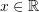that satisfies: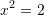In other words, the problem states: “Find a real number such that when it is multiplied by itself, the result is equal to 2”. This problem has led to the development of the modern definition of the set of real numbers consisting of the rational and irrational numbers. The analytical solution for this problem is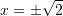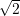is an irrational number. In order to use it for practical or engineering applications, an approximation in the form of a rational number that is “close enough” is used instead. There are various methods to find this rational number approximation of. For example, we will employ the Babylonian method which starts by rearranging the equation as follows: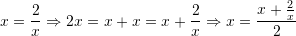The approximation is then found using an iterative method. We first assume an initial value forand designate it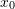. For example, we set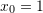. Then, for the iteration number, we calculate a new approximation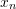by using the formula: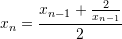So, in the first iteration we have: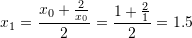Now,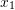is not a very good approximation because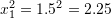. For the next iteration,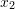can be calculated as: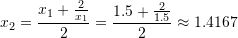is a better approximation because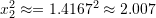. For the next iteration: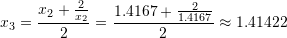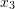is practically equal tobecause when we square it we get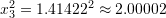! For the sake of completion, let’s try the next iteration: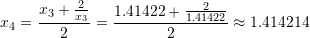For all practical purposes,and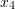are very close to each other (The first four decimal places). The closeness of the two values will be clearly quantified in terms of error measurements in the next section. Once the two values are close up to an acceptable level, we say that convergence has been achieved.